Ecletiwe make software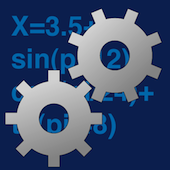iCalcline is analgebra calculator for iOS. It can solve math expression with any level of parenthesis and functions

It can use a table of variables, constant and assign results to values on the fly It manages a table with variables values, using the table values you can write equations as in 'y=x+1' with the 'x' value contained in the table and iCalcline will assign to 'y' the resulting value. In case the y variable was not present when you typed 'y=x+1', a 'y' variable is created for you with a correct value assigned! You can create, manipulate, delete and use how many variables you like inside the app tapping on the variable table.

KEY POINTS

• Evaluates mathematical expressions with parentheses, nested functions, variables and constants • Easy to use • The user simply inserts an expression and iCalcline displays the result • Variable creation and reference inside expressions are very easy using an interactive list.Based on a custom fast calculation engine developed by Tension Software and used also on Mac OS X in Calcline and TS Calc (available on the Mac App Store).Expressions solved optionally step by step showing all the mathematical passages in the log.Variable creation and reference inside expressions are very easy using an interactive list. Variables values are retained over different runs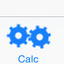The user simply inserts an expression and iCalcline displays the result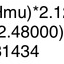Evaluates mathematical expressions with parentheses, nested functions, variables and constantsDEG and RAD calculation for trigonometric functionsUses long double calculation (High precision with up to 9 decimal digit precision)Unbelievable fast even for complex calculationsWe use only Apple native technology to create iOS apps because we love the Apple platform and and we love pure true iOS apps.

FEATURES

• Manages any nested level of parentheses using the correct precedence
• Switch between small stripe / large window
• Offers buttons for fast introduction of any literal values available in the tables (variables) and functions.
• Can change on the fly calculation precision (up to 9 decimals thanks to use of long double calculation)
• Manages unlimited numbers of variables you like in the table.
• Uses decimal separator in accordance with your local settings
• Full log of executed calculations
• Optional step by step solving showing all the mathematical passages in the log.
• Retains values over successive re-launch
• Buttons for fast introduction of variables and functions
• DEG and RAD calculation for trigonometric functions
• Based on a custom fast calculation engine and used also on macOS in Calcline and TS Calc (available on the Mac App Store).
• Uses long double calculation (High precision with up to 9 decimal digit precision)
• Help included
Want to know more details about iCalcline?
For general purpose questions about our product can be also useful to visit the Tension Software Generic FAQ section.
To know what's new from the previous releases visit the iCalcline Releases page.

FUNCTIONS

• sqrt
• cbrt
• sin
• cos
• tan
• asin
• acos
• atan
• sinh
• cosh
• tanh
• asinh
• acosh
• atanh
• log
• log10
• exp,
• exp2
• expm1
• abs
• ceil
• floor
• pow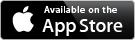iCalcline Rel 1.2.3 (Feb. 13, 2019) - 300 KB Requires: iOS 9 To know what's new from the previous releases CLICK HERE
If you want to know more about how buying works, visit the Buy section of our site
 Copyright © Roberto Panetta. All right reserved. Ecleti di Panetta Salvatore Roberto P.IVA:IT10337860968

iCalcline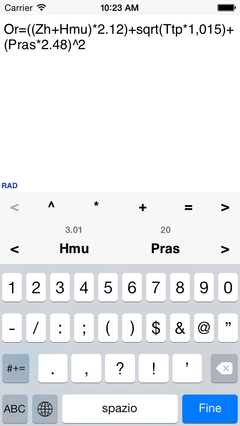×

iCalcline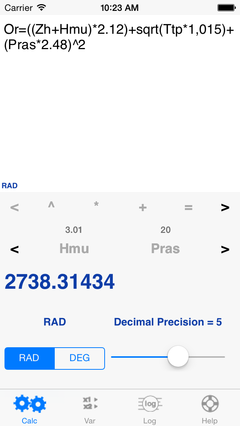×

iCalcline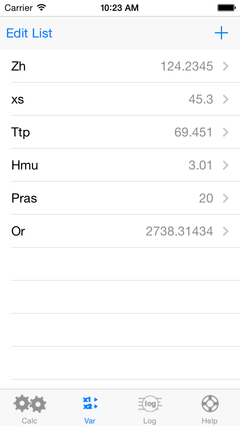×

iCalcline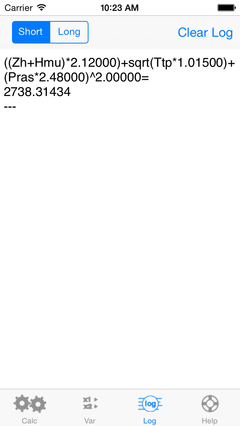×

iCalcline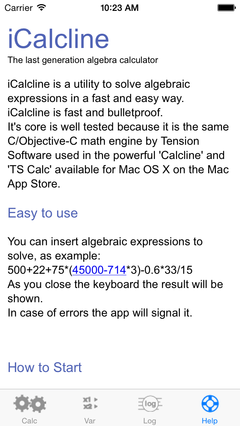×